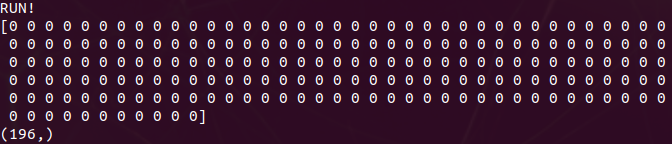# Not sure how to get output of model?

I’m trying to control the steering of a car by getting an output between -1 ,1. Currently, I trained my network with this model.

``````import torch.nn as nn
import torch.nn.functional as F

# define the CNN architecture
class Net(nn.Module):
def __init__(self):
super(Net, self).__init__()
# convolutional layer (sees 32x32x3 image tensor)
self.conv1 = nn.Conv2d(3, 16, 3, padding=1)
# convolutional layer (sees 16x16x16 tensor)
self.conv2 = nn.Conv2d(16, 32, 3, padding=1)
self.pool = nn.MaxPool2d(2, 2)
self.fc1 = nn.Linear(32 * 4 * 4, 256)
self.fc2 = nn.Linear(256, 1)
self.dropout = nn.Dropout(0.25)

def forward(self, x):
x = self.pool(F.relu(self.conv1(x)))
x = self.pool(F.relu(self.conv2(x)))
# flatten image input
x = x.view(-1, 32 * 4 * 4)
x = self.dropout(x)
x = F.relu(self.fc1(x))
x = self.dropout(x)
x = self.fc2(x)
return x

# create a complete CNN
model = Net()
print(model)

# move tensors to GPU if CUDA is available
if train_on_gpu:
model.cuda()
``````

Also you can see the jupyter notebook here The problem is i’m not sure what to use to get the output in real time such as np.argmax, np.max, ect…

``````    def autopilot(self):

img = self.preprocess(self.cam.value)
count = self.cam.count

if count!= self.temp:
print('RUN!')
self.model.eval()
output = self.model(img)
_, angle_tensor = torch.max(output,1)
self.angle_out = angle_tensor.cpu().data.numpy()
#self.angle_out = np.argmax(output.cpu().data.numpy())#angle.cpu().numpy()
self.temp = count
print(self.angle_out)

else:
pass
``````

I’m new to pytorch and i’m stuck trying to get the correct output like I did in jupyter notebook

In teory you are not doing anything wrong.
To get outputs you just have to do the same than for training but adding under the decoration wtih torch.no_grad():

is it not what you tried?
COuld you provide few more details about what does it fail?

The issue that I’m having is that my output is a array that is 195 in length for each image I pass through my model. I just want a single numpy output between -1,1. I think I set up my output function incorrectly. Here is a project very similar to what i’m trying to do https://github.com/autorope/donkeycar/blob/dev/donkeycar/parts/keras.py

But as far as I can see you have a fc layer which goes from 256 to 1 feature. I find it’s not possible you to get 195 elements on a tensor. If you does not modify the network it should be a tensor of size batch x 1 or similar to that.
Could you print output shape? Or, are you running batch size 195 or getting 195 items in the batch dimension when you apply that x = x.view(-1, 32 * 4 * 4)?

Here is my outputThe problem is that your output before applying reshaping is Batchx32x56x56
Why do you reshape that to -1,512?
It makes no sense and that’s why it does not work.

You are squeezing basically maping around 100k elements into a 196*512 matrix

1 Like

Thanks, I never changed the flatten size after modifying a the network. I forgot my input is 244x244 > maxpool > 122x122 > maxpool > 56x56. Then multiplied by out_channel size of 32